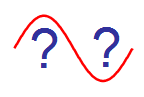Autour Du Signal Sinusoidal

10 Questions | Total Attempts: 351Settings• 1.
Quelle est la fréquence du signal S(t) ?
• A.

100Hz

• B.

50Hz

• C.

• D.

• 2.
Quelle est la valeur efficace d'un signal sinusoïdal dont l'amplitude est de 2Vpp et dont la valeur moyenne est nulle ?
• A.

2V

• B.

1,41V

• C.

0,707V

• D.

2,83V

• 3.
Sur un analyseur de spectre FFT on mesure un niveau de 9dBV pour un signal sinusoidal. Quelle est son amplitude crête ?
• A.

4V

• B.

2,8V

• C.

9V

• D.

0,45V

• 4.
Quel est le niveau en dBV d'un signal sinusoidal d'amplitude 1Vpp ?
• A.

-6dBV

• B.

-9dBV

• C.

-3dBV

• D.

0,5dBV

• 5.
Quelle est l'expression correspondante au signa l sinusoidal représenté ci-dessous ?
• A.
• B.
• C.
• D.
• 6.
Les représentations fréquentielle et temporelle sont-elles cohérentes ?
• A.

Vrai

• B.

Faux

• 7.
Quelle est la pulsation d'un signal sinusoïdal de fréquence 440Hz ?
• A.

• B.

• C.

• D.

• 8.
Quelle est l'amplitude crête à crête du signal sinusoïdal suivant ?
• A.

3V

• B.

1,5V

• C.

2V

• D.

6V

• 9.
Quelle est l'expression du signal dont le spectre est représenté ci-dessous ?
• A.

1+sin(6283t)

• B.

1,41-cos(6283.t)

• C.

1-1,41.cos(6283.t)

• D.

1,41+1,41.sin(6283.t)

• 10.
Quelle est la puissance normalisée d'un signal sinusoidal d'amplitude 4Vpp ?
• A.

4V²

• B.

2V²

• C.

8V²

• D.

2,82V²Back to top